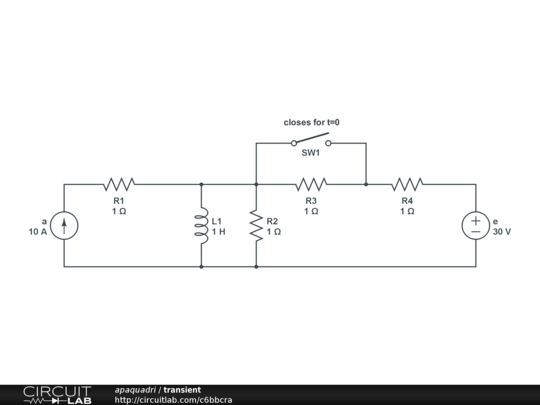# How to solve a transient circuit

Given this circuit considering that before the switch closes the network is in a steady state.

What steps should one follow to trace $V_{L1}$ before and after the closing of the circuit ?

As far as i know there is a transient formula involved $V(t)=[V(0_{+}) - V_{ \infty}]\exp{^{\frac{-t}{\tau}}}+V_{ \infty}$

I had no problems calculating ${\tau}$

What I'm having a hard time with are $V_{ \infty} , V(0_{+})$ and how to trace the graph once I calculated them, could you provide any insights for this ?

In my case is useful to calculate $V(0_{-})$ ?

What would have changed if instead of the inductor I would have had an capacitor ?(Correct me if my thoughts are wrong)

For Olin : I would calculate $R_{eq}=R_{2}//R_{4} = {\frac{1}{2}}$ and having $R_{1}$ in an open circuit provided by $a$ , $R_{2}$ in a short circuit provided by $L_{1}$ and $R_{3}$ in a short circuit provided by $SW1$

So for $t<0$ the tension $V_{0-}=0$ because $L1$ is a short circuit ?

While for $t>0$ one could calculate $V_{0+}$ but here is where I get stuck as in my solution it suggests to use Millman theorem and calculate $V_{0+}={\frac{a-I_{L1(0_{+})}+{\frac{e}{R_{4}}}}{{\frac{1}{R_{2}}}+{\frac{1}{R_{4}}}}}$ In this case what I don't understand is why they consider part of the contribute the current $I_{L1(0_{+})}$ ?

Is $I_{L1(0_{+})}$ same as $I_{L1(0_{-})}$ ? If yes why ?

Since this is a homework problem, I'm not just going to give you the answer. However, consider simplifying the circuit first. You have a current source and a voltage source. Do you remember some techniques for merging them to a single equivalent source with a single resistance? Look up "Thevenin" and "Norton" if not.

Also consider the inductor in steady state. Inductors and capacitors can both be greatly simplified for the purpose of steady state analisys. What is the simplification of a inductor in steady state? Using that, what is the inductor voltage and current in steady state in both cases?

You need to show some work on your own before we can continue and eventually get to the solution.

It seems you have made some correct observations, but I'm not following all you are saying. You are right in that the inductor is a short circuit in steady state.

There are really two parts to this problem. Find the inductor current before the switch closes, then apply that as the initial condition for the circuit with the switch closed. The circuit with the switch open is irrelevant other than to find the inductor current.

For the switch open steady state case, the voltage accross the inductor and R2 is 0. Note that R1 is irrelevant as it is in series with a current source. Since the inductor voltage is 0, the two sources are essentially decoupled and the current contribution from each can be simply added. The current source tells you its current outright, so that's 10 A thru the inductor. The 30 V source has 2 Ω load on it, so that's another 15 A. The inductor current is therefore 25 A. That's the only relevant piece of information from before the switch closes.

For calculating the response, I would first calculate the steady state inductor current with the switch closed. Then, transform the two voltage sources and various resistors into a single Norton or Thevenin source. Actually you only need the resistance of that source. You know the inductor will exponentially decay from its initial value to the steady state value with a time constant of L/R.

So go ahead and perform these simplifications, tell us the final inductor current and the effective impedance it sees. The answer is then just a simple exponential.

You can use Laplace transforms, go into frequency space, find V(s) and everything else you need, come back in time space, find v(t) and everything else you need.

Laplace transforms